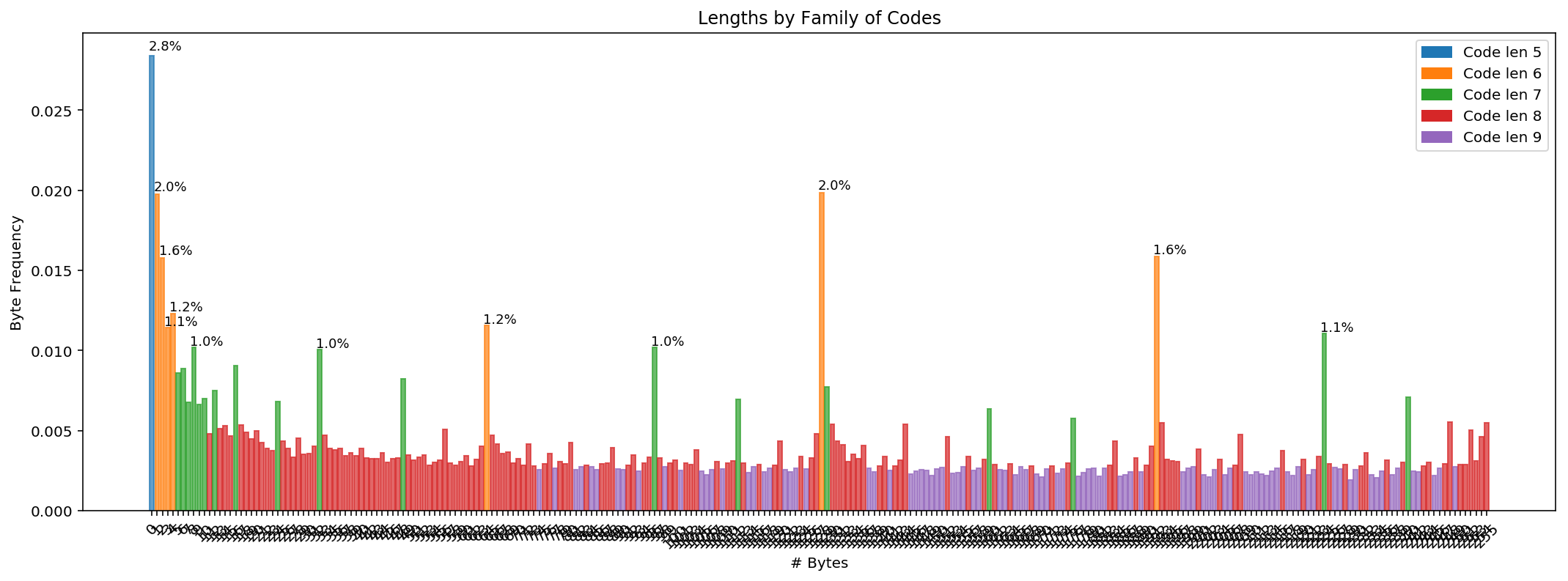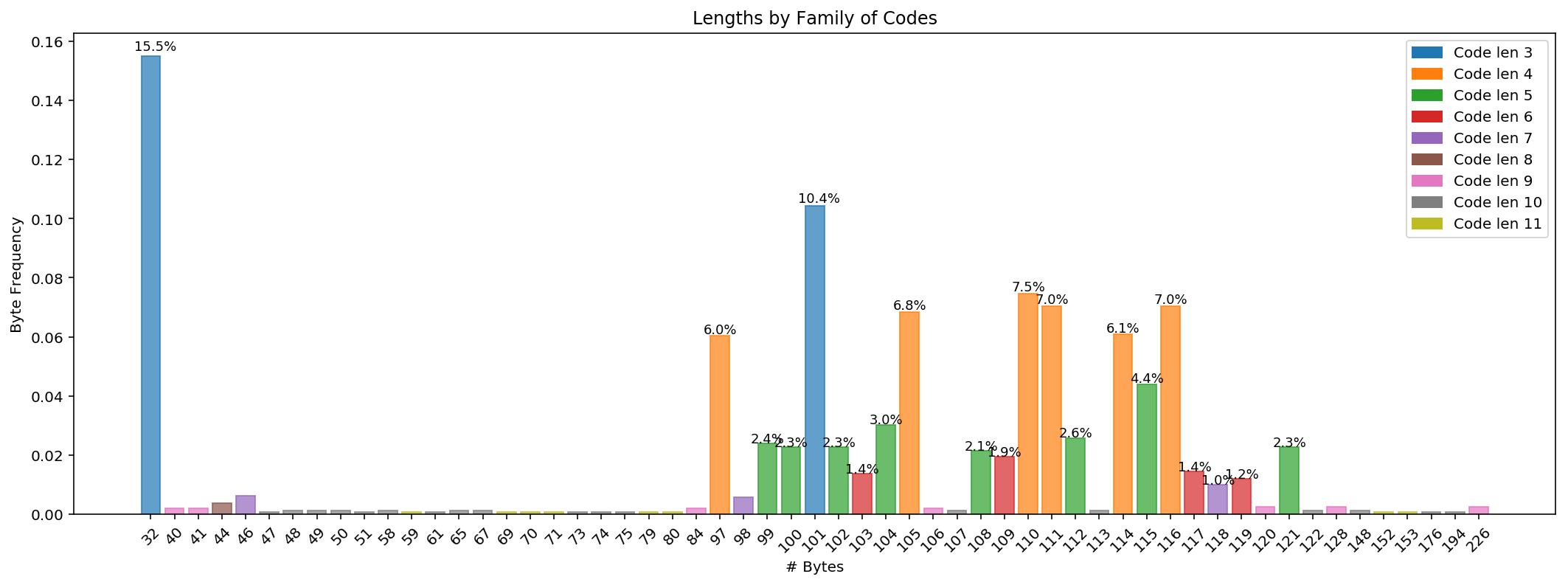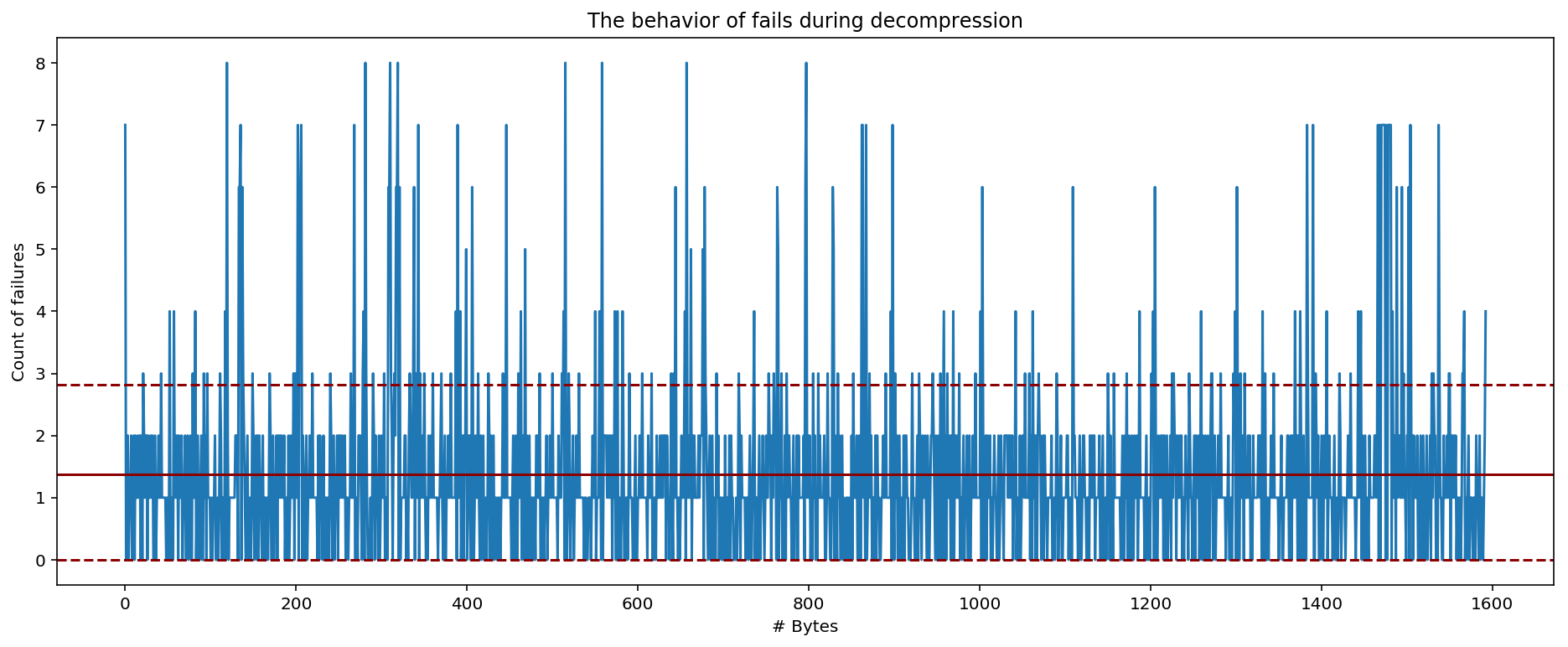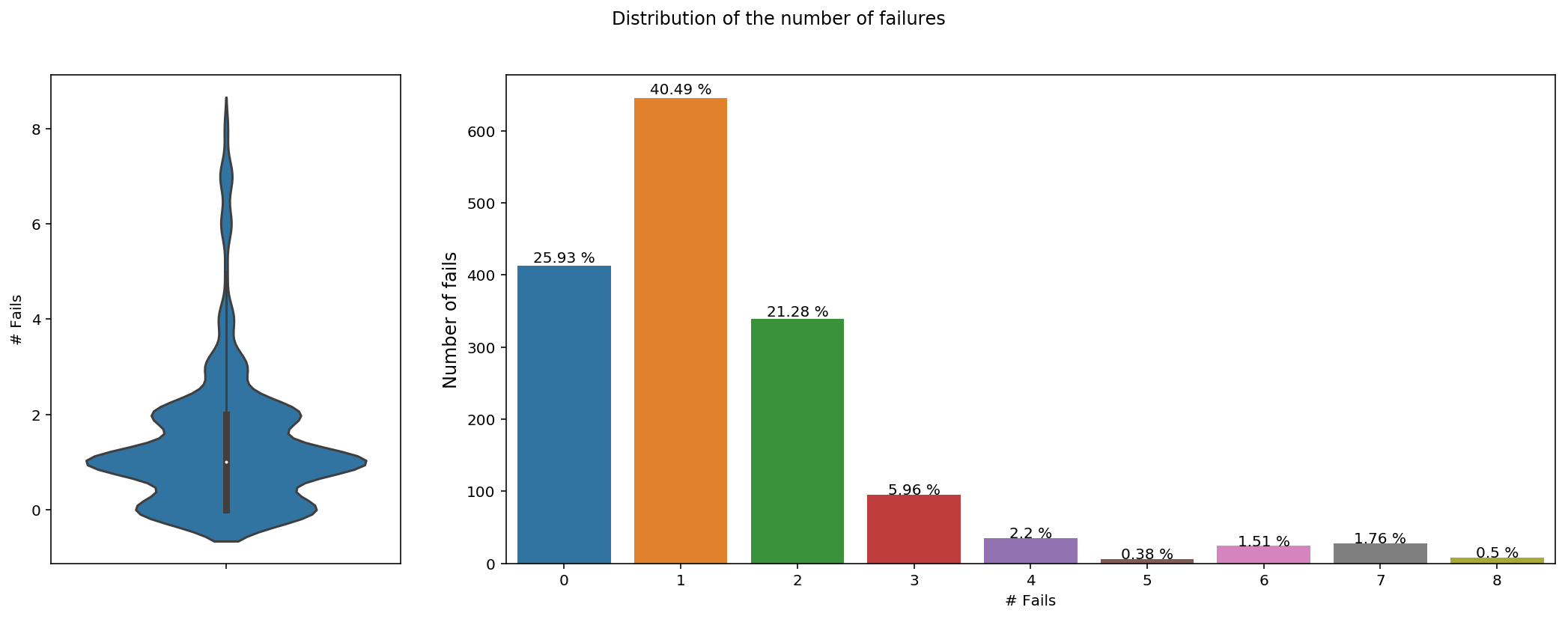# Huffman Coding¶

• Created by Andrés Segura Tinoco
• Created on June 20, 2019

In computer science and information theory, a Huffman Code is a particular type of optimal prefix code that is commonly used for lossless data compression. The output from Huffman's algorithm can be viewed as a variable-length code table for encoding a source symbol (such as a character in a file). The algorithm derives this table from the estimated probability or frequency of occurrence (weight) for each possible value of the source symbol. 

In :
# Load Python libraries
import numpy as np
import timeit
import pandas as pd
import statistics as stats
from collections import Counter

In :
# Load Plot libraries
import seaborn as sns
import matplotlib.pyplot as plt
import matplotlib.patches as mpatches
from matplotlib import gridspec
%matplotlib inline
%config InlineBackend.figure_format = 'retina'


## 1. Huffman Code from Scratch¶

Huffman coding uses a specific method for choosing the representation for each symbol, resulting in a prefix code (sometimes called "prefix-free codes", that is, the bit string representing some particular symbol is never a prefix of the bit string representing any other symbol). Huffman coding is such a widespread method for creating prefix codes that the term "Huffman code" is widely used as a synonym for "prefix code" even when such a code is not produced by Huffman's algorithm. 

In :
# Class HuffmanCode from scratch
class HuffmanCode:

# Return a Huffman code for an ensemble with distribution p
def get_code(self, p_symbols):

# Init validation
n = len(p_symbols)
if n == 0:
return dict()
elif n == 1:
return dict(zip(p_symbols.keys(), ['1']))

# Ensure probabilities sum to 1
self._normalize_weights(p_symbols)

# Returns Huffman code
return self._get_code(p_symbols);

# (Private) Calculate Huffman code
def _get_code(self, p):

# Base case of only two symbols, assign 0 or 1 arbitrarily
if len(p) == 2:
return dict(zip(p.keys(), ['0', '1']))

# Create a new distribution by merging lowest prob pair
p_prime = p.copy()
s1, s2 = self._get_lowest_prob_pair(p)
p1, p2 = p_prime.pop(s1), p_prime.pop(s2)
p_prime[s1 + s2] = p1 + p2

# Recurse and construct code on new distribution
code = self._get_code(p_prime)
symbol = s1 + s2
s1s2 = code.pop(symbol)
code[s1], code[s2] = s1s2 + '0', s1s2 + '1'

return code;

# (Private) Return pair of symbols from distribution p with lowest probabilities
def _get_lowest_prob_pair(self, p):

# Ensure there are at least 2 symbols in the dist.
if len(p) >= 2:
sorted_p = sorted(p.items(), key=lambda x: x)
return sorted_p, sorted_p;

return (None, None);

# (Private) Makes sure all weights add up to 1
def _normalize_weights(self, p_symbols, t_weight=1.0):
n = sum(p_symbols.values())

if n != t_weight:
for s in p_symbols:
p_symbols[s] = p_symbols[s] / n;


Input: $$A = \{ a_1, a_2, a_3, ..., a_n \} \tag{1}$$

$$W = \{ w_1, w_2, w_3, ..., w_n \} \tag{2}$$
$$n = |A|$$

Output: $$C(A, W) = \{ c_1, c_2, c_3, ..., c_n \} \tag{3}$$

Target: $$L(C) = \sum_{i=1}^n{w_i . length(c_i)} \tag{4}$$

$$L(C) < L(T)\;for\;any\;code\;T(A, W)$$
In :
# Create Huffman Code instance
hc = HuffmanCode()


### Simple Examples¶

In :
# Alphabet with 1 symbol
sample_1 = { 'a': 1.0 }
hc.get_code(sample_1)

Out:
{'a': '1'}
In :
# Alphabet with 3 symbols and total probability less than 1
sample_2 = { 'a': 0.6, 'b': 0.25, 'c': 0.1 }
hc.get_code(sample_2)

Out:
{'a': '0', 'c': '10', 'b': '11'}
In :
# Alphabet with 5 symbols and total probability equal than 1.0
sample_3 = { 'a': 0.10, 'b': 0.15, 'c': 0.30, 'd': 0.16, 'e': 0.29 }
hc.get_code(sample_3)

Out:
{'e': '10', 'c': '11', 'd': '00', 'a': '010', 'b': '011'}

## 2. Compress Image with Huffman Code¶

This example is with a PNG image. Experimentally it was found that the distribution of the bytes of an image tends to be uniform.

In :
# Read file in low level (Bytes)
def get_file_bytes(file_path):
with open(file_path, 'rb') as f:
return None;

In :
# Loading target image
file_path = "../data/img/example-3.png"
image_byte_list = get_file_bytes(file_path)

In :
# Calculate code frequency
def get_term_freq(term_list):
term_freq = {}
terms_count = dict(Counter(term_list))

for key, value in terms_count.items():
if isinstance(key, int):
key = str(key)
term_freq[key] = value

return term_freq;

In :
# Alphabet with 256 symbols
term_freq = get_term_freq(image_byte_list)
len(term_freq)

Out:
256
In :
# Normalize term frequency
n = sum(term_freq.values())
for term in term_freq:
term_freq[term] = term_freq[term] / n;
sum(term_freq.values())

Out:
0.9999999999999994
In :
# Get Huffman coding
hf_code = hc.get_code(term_freq)

In :
# Creates a huffman dataframe with the codes and frequency of each of them
def create_huffman_df(code_list, term_freq):
codes = pd.DataFrame([code_list, term_freq]).T
codes.reset_index(level=0, inplace=True)
codes.columns = ["byte", "code", "frequency"]
codes['symbol'] = [chr(int(b)) for b in codes["byte"]]
codes = codes[["byte", "symbol", "code", "frequency"]]

return codes

In :
# Create and showing data
codes = create_huffman_df(hf_code, term_freq)

Out:
byte symbol code frequency
0 0 01110 0.0284127
1 1  110011 0.0197444
2 10 \n 0110101 0.00697332
3 100 d 01000010 0.00314809
4 101 e 110101111 0.0025088
5 102 f 00101111 0.00298616
6 103 g 00011000 0.0028726
7 104 h 10000101 0.00378738
8 105 i 110010111 0.00247305
9 106 j 101101001 0.00226065
10 107 k 111000010 0.00255716
11 108 l 00110110 0.00307028
12 109 m 111010011 0.00260974
13 11 11000010 0.00477786
14 110 n 00101001 0.00295672
15 111 o 00111110 0.00311865
16 112 p 0110011 0.00692705
17 113 q 00101010 0.00296303
18 114 r 110000000 0.00237421
19 115 s 111110101 0.00275694
In :
# Save full huffman codes as CSV file
codes.to_csv('../huffman_codes/sample1.csv', index=False)

In :
# Calculate message average size
msg_size_current = 8
msg_size_weighted = 0

for key, value in hf_code.items():
msg_size_weighted += len(value) * term_freq[key]

In :
# Current message average size (bits per symbol)
msg_size_current

Out:
8
In :
# Weighted message average size (bits per symbol)
msg_size_weighted

Out:
7.783808280076634

### Real compression percentage¶

In :
# Calculating compression ratio (%)
compress_rate = (msg_size_current - msg_size_weighted) / msg_size_current
print(round(compress_rate * 100, 2), '%')

2.7 %


Main function: compress a binary file using a huffman code

In :
# Compress a binary file using a huffman code
def compress_bin_file(byte_list, code_list):
bits_string = ''
compress_list = []
symbols_used = {}

for symbol in byte_list:
key = str(symbol)
new_symbol = code_list[key]
compress_list.append(new_symbol)

# Save frequency of length of used symbols
sym_len = len(new_symbol)
if sym_len in symbols_used:
symbols_used[sym_len] += 1
else:
symbols_used[sym_len] = 1

# Create bits string
bits_string = "".join(compress_list)

# Sort dict by key
symbols_used = sorted(symbols_used.items(), key=lambda x: x)

# Return compressed file and used symbols
return bits_string, dict(symbols_used)

In :
# Compressing PNG image with Huffman code
compress_file, symbols_used = compress_bin_file(image_byte_list, hf_code)
print(compress_file[:508])

1110101111010001000110101000010111110010001101011000101001101010111001110011101110010000000100100111110111111111111101101110011100110101011100111001110101011100011111100010001000111001110011101111010011000000011010100111001000001110011100111000111000011000101111101111000011011111001110011101010011010100000011000010110110100101010010100100111001110011101100111111101011111110110000101110100000011101110100101111000001011010110110000001110011100001000111010100010011110101111100010010101111110011001100111010

In :
# Weight of the compressed PNG image (KB)
print(round(len(compress_file) / 8 / 1024, 2), 'KB')

451.83 KB

In :
# Weight of the original PNG image (KB)
print(round(len(image_byte_list) / 1024, 2), 'KB')

464.38 KB


### Lengths by Family of Codes¶

The code family that encoded each byte is plotted below.

In :
# Function that plots the bytes encoded by a family type by length
def plot_lens_by_codes_families(term_freq, hf_code):
# Set up the matplotlib figure
fig, ax = plt.subplots(figsize = (18, 6))

bars = []
values= []
for ix in range(256):
key = str(ix)
if key in term_freq.keys():
bars.append(key)
values.append(term_freq[key])
y_pos = np.arange(len(bars))

# Vertical barchart
barlist = plt.bar(y_pos, values, alpha = 0.7)
plt.xticks(y_pos, bars, rotation=45)

# Add bar labels
lens = []
for i, v in enumerate(values):
key = str(bars[i])
length = len(hf_code[key])
l = str(round(v * 100, 1)) + '%'
if v > 0.01:
ax.text((i - 0.7), (v * 1.0125), str(l), color = "black", fontweight = "normal", fontsize = 9)
barlist[i].set_color(palette[str(length)])

# Add custom legend
legend_list = []
for k, v in palette.items():
legend_list.append(mpatches.Patch(color = v, label = 'Code len ' + k))

plt.legend(handles = legend_list)
plt.ylabel('Byte Frequency')
plt.xlabel('# Bytes')
plt.title('Lengths by Family of Codes')
plt.show()

In :
# Show frequency of length of used symbols
symbols_used

Out:
{5: 13511, 6: 50643, 7: 68876, 8: 234607, 9: 107890}
In :
# Palette of colores
palette = {"5": "#1f77b4", "6": "#ff7f0e", "7": "#2ca02c", "8": "#d62728", "9": "#9467bd"}

In :
# Create plot
plot_lens_by_codes_families(term_freq, hf_code)## 3. Compress Text file with Huffman Code¶

In this example, the compression and decompression functions will be tested with the abstract of an English paper.

In :
# Loading target text book
file_path = "../data/text/abstract1-en.txt"
text_byte_list = get_file_bytes(file_path)

In :
# Alphabet with 256 symbols
term_freq = get_term_freq(text_byte_list)
len(term_freq)

Out:
57
In :
# Normalize term frequency
n = sum(term_freq.values())
for term in term_freq:
term_freq[term] = term_freq[term] / n;
sum(term_freq.values())

Out:
1.0000000000000004
In :
# Get Huffman coding
hf_code = hc.get_code(term_freq)

In :
# Create and showing the Huffman codes
codes = create_huffman_df(hf_code, term_freq)

Out:
byte symbol code frequency
0 100 d 00010 0.0225989
1 101 e 010 0.104206
2 102 f 00011 0.0225989
3 103 g 001110 0.0138104
4 104 h 10000 0.0301318
5 105 i 1001 0.0684244
6 106 j 001111110 0.00188324
7 107 k 1111010100 0.00125549
8 108 l 00000 0.0213434
9 109 m 111100 0.0194601
10 110 n 1110 0.0747018
11 111 o 1011 0.0703076
12 112 p 00110 0.0257376
13 113 q 1111011001 0.00125549
14 114 r 0111 0.0608914
15 115 s 11111 0.0439422
16 116 t 1010 0.0703076
17 117 u 100010 0.0144382
18 118 v 1000111 0.0100439
19 119 w 001001 0.0119272
In :
# Save full huffman codes as CSV file
codes.to_csv('../huffman_codes/sample2.csv', index=False)

In :
# Calculate weighted message size average
msg_size_current = 8
msg_size_weighted = 0

for key, value in hf_code.items():
msg_size_weighted += len(value) * term_freq[key]

In :
# Current message size average (bits per symbol)
msg_size_current

Out:
8
In :
# Weighted message size average (bits per symbol)
msg_size_weighted

Out:
4.369742623979907

### Real compression percentage¶

In :
# Calculating compression ratio (%)
compress_rate = (msg_size_current - msg_size_weighted) / msg_size_current
print(round(compress_rate * 100, 2), '%')

45.38 %

In :
# Compressing text file with Huffman code
compress_file, symbols_used = compress_bin_file(text_byte_list, hf_code)
print(compress_file[:508])

1111010010110101010000010101101110000111010011111111000110011110110011010111111101000010110001001100001001001011000011000101101111101111110010001001001111111111010011110000111011011111110001101010100110111110110101010111100010001010110011011000100010110111111001001011100011001111011001100100111101000001110101100011110101010000010110100010111010011000111010011111111010110101010000010110001001011000001110111100011010101010010011111001101110000101100101110010011100111000001110011001110100011110110111101110

In :
# Weight of the compressed text file (KB)
print(round(len(compress_file) / 8 / 1024, 2), 'KB')

0.85 KB

In :
# Weight of the original text file (KB)
print(round(len(text_byte_list) / 1024, 2), 'KB')

1.56 KB


### Lengths by Family of Codes¶

The code family that encoded each byte is plotted below.

In :
# Show frequency of length of used symbols
symbols_used

Out:
{3: 413, 4: 645, 5: 339, 6: 95, 7: 35, 8: 6, 9: 24, 10: 28, 11: 8}
In :
# Palette of colores
palette = {"3": "#1f77b4", "4": "#ff7f0e", "5": "#2ca02c", "6": "#d62728", "7": "#9467bd",
"8": "#8c564b", "9": "#e377c2", "10": "#7f7f7f", "11": "#bcbd22"}

In :
# Create plot
plot_lens_by_codes_families(term_freq, hf_code)## 4. Decompress file with Huffman Code¶

### 4.1. Simple approach¶

Test with all huffman codes until the file is completely decompressed. The codes are sorted ascending by their length.

In :
# Creates a dataframe with the codes, frequency and compression percentage of each of them
def create_codes_df(code_list, hf_code, col_sort):
term_freq = Counter([len(v) for k, v in hf_code.items()])
df_codes = pd.DataFrame([code_list, term_freq]).T
df_codes.reset_index(level=0, inplace=True)
df_codes.columns = ["code_size", "bytes_compressed", "code_count"]
df_codes['code_comp_perc'] = round(df_codes.bytes_compressed / df_codes.code_count, 2)
df_codes = df_codes[["code_size", "code_count", "bytes_compressed", "code_comp_perc"]]

return df_codes.sort_values(by=[col_sort], ascending=False)

In :
# Show frequency of length of used symbols
df = create_codes_df(symbols_used, hf_code, 'code_comp_perc')
df.style.set_table_styles([
{'selector': '.row_heading, .blank', 'props': [('display', 'none;')]}
])

Out:
code_size code_count bytes_compressed code_comp_perc
0 3 2 413 206.5
1 4 6 645 107.5
2 5 8 339 42.38
3 6 4 95 23.75
4 7 3 35 11.67
5 8 1 6 6
6 9 7 24 3.43
7 10 18 28 1.56
8 11 8 8 1
In :
# Decompress a binary file using a huffman code
def decompress_bin_file(byte_string, hf_code, symb_used):
start_time = timeit.default_timer()
byte_list = []
codes_size = []
inv_codes = {v: k for k, v in hf_code.items()}
fails_list = []
n_size = len(byte_string)

# Sort codes_size list by code length
for k, v in symb_used.items():
codes_size.append(k)
codes_size.sort(reverse = False)

# Decompress
ix = 0
while ix < n_size:
curr_tries = 0

for size in codes_size:
possible_code = byte_string[ix:ix + size]

if possible_code in inv_codes.keys():
byte = int(inv_codes[possible_code])
byte_list.append(byte)
ix = ix + size
fails_list.append(curr_tries)
break
curr_tries += 1

# Elapsed time
elapsed = timeit.default_timer() - start_time

# Algorithm accuracy
total_tries = sum(fails_list)
algo_accuracy = round(100 * len(byte_list) / total_tries, 2)

# Verbose
print(codes_size)
print('elapsed time', elapsed, 's')
print('tries:', total_tries, ', acurracy:', algo_accuracy, '%')

return byte_list, fails_list, codes_size;

In :
# Decode/Decompress file using the Huffman code
decompress_file, fails_list, codes_size = decompress_bin_file(compress_file, hf_code, symbols_used)

[3, 4, 5, 6, 7, 8, 9, 10, 11]
elapsed time 0.0041066 s
tries: 2182 , acurracy: 73.01 %

In :
# Weight of the original text file (KB)
print(round(len(decompress_file) / 1024, 2), 'KB')

1.56 KB


#### Comparing if the original file and the decompressed file are the same (equals)¶

In :
# Compare if two files are equals for equality and element-wise
def compare_files(file_a, file_b):
return np.array_equiv(file_a, file_b)

In :
# Comparing files
compare_files(text_byte_list, decompress_file)

Out:
True

#### Show fails behavior during decompression¶

In :
# Function that plots the fails behavior of the decompression process
def plot_fails_behavior(f_data):

# Average failures per decompressed byte
f_mean = stats.mean(f_data)
f_stdev = stats.pstdev(f_data)
print(round(f_mean, 4), 'fails/byte with a std dev:', round(f_stdev, 4))

max_value = max(f_data)
min_value = 0
upper_lim = min(max_value, (f_mean + f_stdev))
lower_lim = max(min_value, (f_mean - f_stdev))

fig = plt.figure(figsize = (16, 6))
plt.plot(f_data)
plt.axhline(y = upper_lim, color = "#8b0000", linestyle = "--")
plt.axhline(y = f_mean, color = "#8b0000", linestyle = "-")
plt.axhline(y = lower_lim, color = "#8b0000", linestyle = "--")
plt.title('The behavior of fails during decompression')
plt.xlabel('# Bytes', fontsize = 10)
plt.ylabel('Count of failures', fontsize = 10)
plt.show()

In :
# Plotting the fails behavior
plot_fails_behavior(fails_list)

1.3697 fails/byte with a std dev: 1.4448In :
# Function that calculates distribution of the number of fails
def calc_fails_dist(f_data):
fails_dist = Counter(f_data)
df_fails_dist = pd.DataFrame.from_records(fails_dist.most_common(), columns = ['n_fails', 'quantity'])
df_fails_dist["fails_perc"] = 100 * df_fails_dist.quantity / len(fails_list)
df_fails_dist = df_fails_dist.sort_values(by=['n_fails'])
return df_fails_dist

In :
# Calculate distribution of the number of fails
df_fails_dist = calc_fails_dist(fails_list)
df_fails_dist

Out:
n_fails quantity fails_perc
1 0 413 25.925926
0 1 645 40.489642
2 2 339 21.280603
3 3 95 5.963591
4 4 35 2.197112
8 5 6 0.376648
6 6 24 1.506591
5 7 28 1.757690
7 8 8 0.502197
In :
# Function that plots the distribution of fails
def plot_fails_dist(f_list, df_f_dist, c_sizes):
fig = plt.figure(figsize = (18, 6))
fig.subplots_adjust(hspace = 0.15, wspace = 0.15)
gs = gridspec.GridSpec(1, 2, width_ratios=[1, 3])

ax0 = plt.subplot(gs)
sns.violinplot(y=f_list)
ax0.set_ylabel('# Fails', fontsize = 10)

colors = []
for size in c_sizes:
colors.append(palette[str(size)])

ax1 = plt.subplot(gs)
g = sns.barplot(x = 'n_fails', y = 'quantity', data = df_f_dist, palette=colors)
for index, row in df_f_dist.iterrows():
lbl_value = str(round(row.fails_perc, 2)) + ' %'
g.text(row.n_fails, row.quantity * 1.01, lbl_value, color='black', ha="center")
ax1.set_xlabel('# Fails', fontsize = 10)
ax1.set_ylabel('Number of fails', fontsize = 12)

fig.suptitle('Distribution of the number of failures')
plt.show()

In :
# Plotting the distribution of fails
plot_fails_dist(fails_list, df_fails_dist, codes_size)### 4.2. Probabilistic approach¶

Based on the probability of occurrence of each family of symbols...

In :
# Sort dict by probability of occurrence
symbols_used_sort = dict(sorted(symbols_used.items(), key=lambda kv: kv, reverse=True))

In :
# Show frequency of length of used symbols
df = create_codes_df(symbols_used_sort, hf_code, 'bytes_compressed')
df.style.set_table_styles([
{'selector': '.row_heading, .blank', 'props': [('display', 'none;')]}
])

Out:
code_size code_count bytes_compressed code_comp_perc
1 4 6 645 107.5
0 3 2 413 206.5
2 5 8 339 42.38
3 6 4 95 23.75
4 7 3 35 11.67
7 10 18 28 1.56
6 9 7 24 3.43
8 11 8 8 1
5 8 1 6 6
In :
# Decompress a binary file using a huffman code
def decompress_bin_file_prob(byte_string, hf_code, symb_used):
start_time = timeit.default_timer()
byte_list = []
codes_size = []
inv_codes = {v: k for k, v in hf_code.items()}
fails_list = []
n_size = len(byte_string)

# Sort codes_size list by the probability of the code family
prob_symbols = sorted(symb_used.items(), key=lambda kv: kv, reverse=True)
for k, v in prob_symbols:
codes_size.append(k)

# Decompress
ix = 0
while ix < n_size:
curr_tries = 0

for size in codes_size:
possible_code = byte_string[ix:ix + size]

if possible_code in inv_codes.keys():
byte = int(inv_codes[possible_code])
byte_list.append(byte)
ix = ix + size
fails_list.append(curr_tries)
break
curr_tries += 1

# Elapsed time
elapsed = timeit.default_timer() - start_time

# Algorithm accuracy
total_tries = sum(fails_list)
algo_accuracy = round(100 * len(byte_list) / total_tries, 2)

# Verbose
print(codes_size)
print('elapsed time', elapsed, 's')
print('tries:', total_tries, ', acurracy:', algo_accuracy, '%')

return byte_list, fails_list, codes_size;

In :
# Decode/Decompress file using the Huffman code
decompress_file2, fails_list2, codes_size2 = decompress_bin_file_prob(compress_file, hf_code, symbols_used)

[4, 3, 5, 6, 7, 10, 9, 11, 8]
elapsed time 0.004494800000000021 s
tries: 1904 , acurracy: 83.67 %

In :
# Weight of the original text file (KB)
print(round(len(decompress_file2) / 1024, 2), 'KB')

1.56 KB

In :
# Comparing files
compare_files(text_byte_list, decompress_file2)

Out:
True

#### Show fails behavior during decompression¶

In :
# Plotting the fails behavior
plot_fails_behavior(fails_list2)

1.1952 fails/byte with a std dev: 1.428